This week was my Tidy Tuesday debut! Tidy Tuesday is a weekly data project which is aimed at R users who want to practice their wrangling and visualisation skills within the `tidyverse`. This week’s data set features a historical record of rocket launches, and formed the basis for the article “The space race is dominated by new contenders”.

My goals for this week were to:

• Expose myself to `gganimate`, a really nifty package that extends `ggplot2` to include animations.
• Practice tidy evaluation by building a few reusable functions for plotting.

I won’t focus on the details of the data wrangling in this post. I recommend you watch David Robinson’s excellent Tidy Tuesday screencast, where I picked up some cool tricks. One that I would like to highlight, and that I will definitely add to my toolbox, is the use of `fct_collapse` and `fct_lump` from the `forcats` package. It allows us to easily collapse several factors into manually defined groups. To see how this works, first consider the unprocessed data:

``````library(tidyverse)
library(gganimate)
library(countrycode)
library(knitr)
library(kableExtra)

dir <- "/Users/sean/Documents/spacerace/"
launches_csv <- "launches.csv"

launch_data %>%
count(state_code, sort = TRUE) %>%
kable() %>%
kable_styling(bootstrap_options = c("striped", "condensed"))``````
state_code n
SU 2444
US 1716
RU 734
CN 302
F 291
J 115
IN 65
I-ESA 13
IL 10
I 9
IR 8
KP 5
CYM 4
I-ELDO 3
KR 3
BR 2
UK 2

The table above shows the number of launches by nation. Some of these are actually not nations (such as I-ESA, which represents the European Space Agency). We will deal with these shortly. Given the table above, we would like to:

1. Collapse SU (Soviet Union) and RU (Russia) into one factor.
2. Correct the state abbreviations so that we can conveniently fetch state names using the `countrycode` package.
3. Keep the top six nations, and lump the rest into a group called `Other`.
4. Deal with missing values resulting from abbreviations which do not correspond to a country in step 2.

The following chunk shows how to do all of the above:

``````processed <- launch_data %>%
mutate(state_code_cleaned = fct_collapse(
state_code,
"RU" = c("RU", "SU"), # Collapsing SU and RU into a single factor RU
"FR" ="F",
"JP" = "J",
"IT" = "I")) %>%
mutate(state_name = countrycode( # Using countrycode to obtain state names
state_code_cleaned,
"iso2c",
"country.name")) %>%
mutate(state_name = fct_lump(state_name, 6)) %>% # Lumping factors not in top six into "Other"
replace_na(list(state_name = "Other")) # Dealing with names (e.g. I-ESA) that countrycode can't handle. Since these are not in the top six we simply assign them to "Other".

processed %>%
count(state_name, sort = TRUE) %>%
kable() %>%
kable_styling(bootstrap_options = c("striped", "condensed"))``````
state_name n
Russia 3178
United States 1716
China 302
France 291
Japan 115
India 65
Other 59

Pretty cool! Anyway, on to the visualisations. Following Robinson’s screencast, I ended up with the following plot:

``````processed %>%
count(state_name, launch_year) %>%
ggplot(aes(x = launch_year, y = n, group = state_name)) +
geom_line(aes(color = state_name)) +
labs(title = "Number of yearly launches", x = "Year", y = "Launches") +
theme_minimal() +
theme(legend.title = element_blank())``````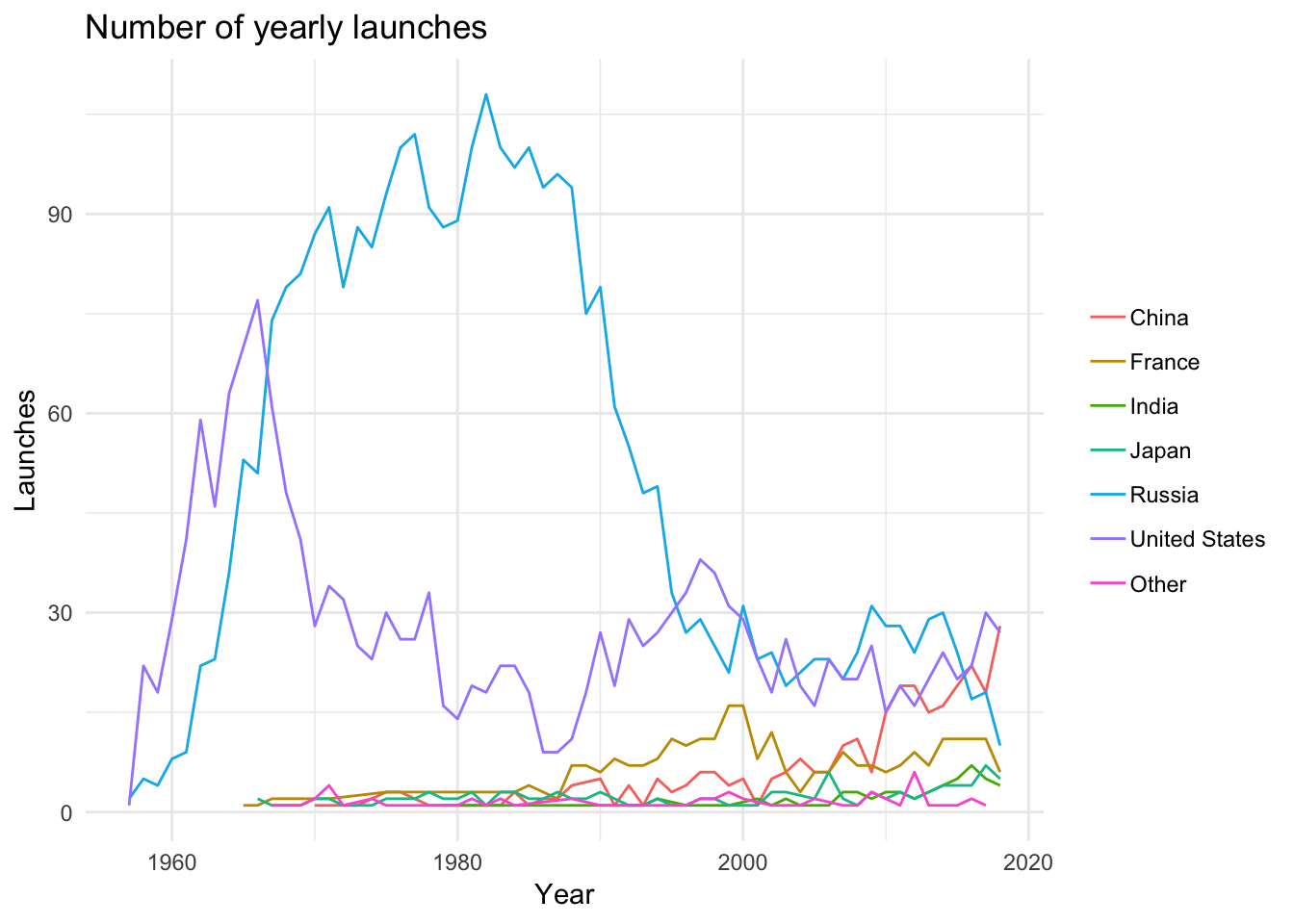The next step was to use `gganimate` to liven things up a bit. Following the time series example from the `gganimate` documentation, I ended up with the following:

``````processed %>%
count(agency_type, launch_year) %>%
ggplot(aes(x = launch_year, y = n, group = agency_type)) +
geom_line(aes(color = agency_type), show.legend = FALSE) +
geom_segment(aes(xend = 2020, yend = n), linetype = 2, colour = 'grey') +
geom_point(aes(color = agency_type), show.legend = FALSE, size = 2) +
geom_text(aes(x = 2021, color = agency_type, label = agency_type), show.legend = FALSE, hjust = 0) +
transition_reveal(launch_year) +
coord_cartesian(clip = 'off') +
labs(title = "Number of yearly launches", x = "Year", y = "Launches") +
theme_minimal() +
theme(plot.margin = margin(5.5, 40, 5.5, 5.5))``````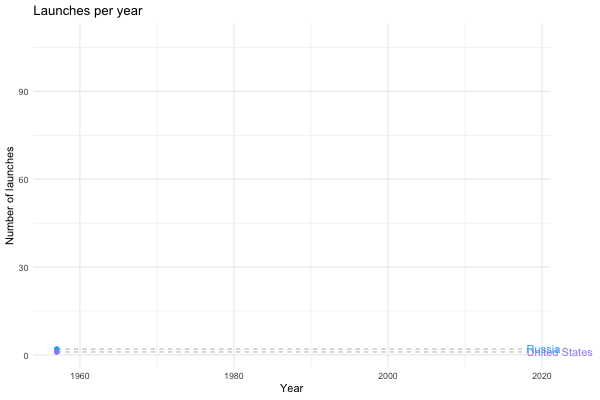Now we’re talking! Next, I wanted to define a relatively general function that allowed me to use the same framework to visualise the growing influence of the private sector in space travel. I would also like the option to set a lower bound for time period I’m interested in, and I would like to be able to specify which countries I’m interested in plotting. Using tidy evaluation, I ended up with the following:

``````launches_per_year <- function(df, variable, countries = c(), from.year = NA, size.margin = 50){

var <- enquo(variable)

if(length(countries) != 0) df <- df %>% filter(state_name %in% countries)
if(!is.na(from.year)) df <- df %>% filter(launch_year >= from.year)

p <- df %>%
count(!! var, launch_year) %>%
ggplot(aes(x = launch_year, y = n, group = !! var)) +
geom_line(aes(color = !! var)) +
geom_segment(aes(xend = max(launch_year), yend = n), linetype = 2, colour = 'grey') +
geom_point(aes(color = !! var), size = 2) +
geom_text(aes(x = max(launch_year), color = !! var, label = !!  var), hjust = 0) +
transition_reveal(launch_year) +
coord_cartesian(clip = 'off') +
labs(title = "Number of yearly launches", x = "Year", y = "Launches") +
theme_minimal() +
theme(plot.margin = margin(t = 5.5, b = 5.5, r = size.margin, l = 5.5),
legend.position = "none")

return(p)
}``````

Now I can call `launches_per_year` with different arguments to generate different plots. For instance, we can see how the private sector gets increasingly involved in space flight…

``launches_per_year(processed, agency_type)``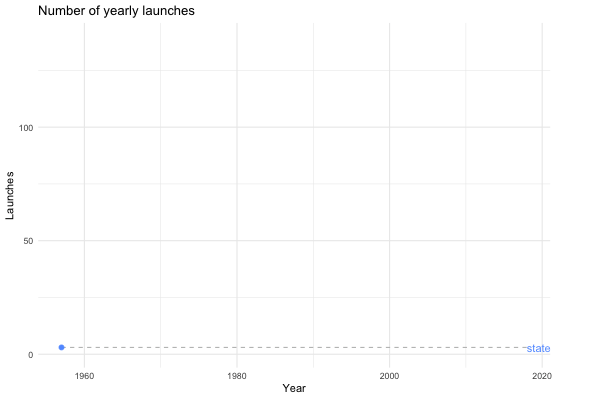… or we could look at the same plot for the last 20 years instead.

``launches_per_year(processed, agency_type, from.year = 2000)``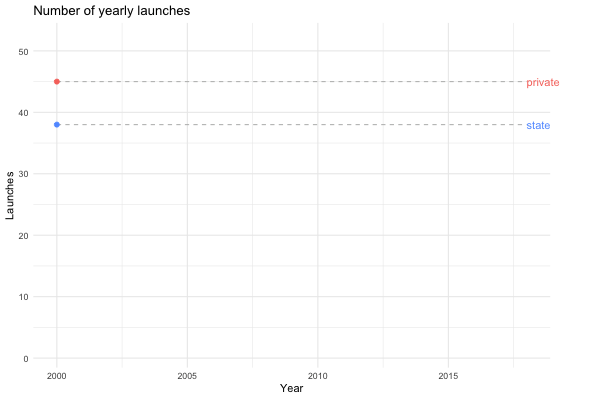Maybe we’re interested in visualising how China has become a spacefaring nation to be reckoned with…

``launches_per_year(processed, state_name, countries = c("United States", "Russia", "China"), from.year = 1990)``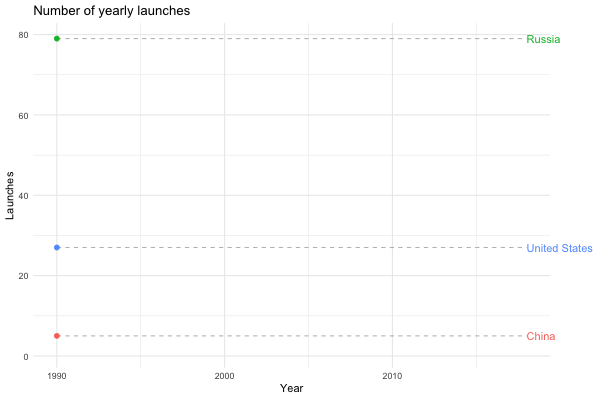I was also interested in the yearly success rates of nations and agency types (public/private/startups). Again, building a relatively general function allows me to explore different options:

``````learning_to_fly <- function(df, variable, names = c()){

var <- enquo(variable)

if(length(names) != 0) df <- df %>% filter(!! var %in% names)
print(dim(df))

p <- df %>%
ggplot(aes(x = launch_year, y = success_rate, group = !! var)) +
geom_line(aes(color = !! var), show.legend = FALSE) +
geom_point(aes(color = !! var, size = number_launches)) +
transition_reveal(launch_year) +
labs(title = "Yearly success rate of rocket launches",
x = "Year",
y = "Successful launches (%)",
size = "",
color = "") +
theme_minimal() +
theme(plot.margin = margin(t = 5.5, b = 5.5, r = 50, l = 5.5),
legend.position = "bottom",
legend.text = element_text(size = rel(1.1)))

return(p)
}``````

For example, if I want to plot the learning curves (if you will) of the American and Soviet/Russian space programs, I can do the following:

``````success_rates <- processed %>%
group_by(state_name, launch_year) %>%
summarise(success_rate = 100 * sum(category == "O") / (sum(category == "O") + sum(category == "F")),
number_launches = n())

learning_to_fly(success_rates, state_name, names = c("Russia", "United States"))``````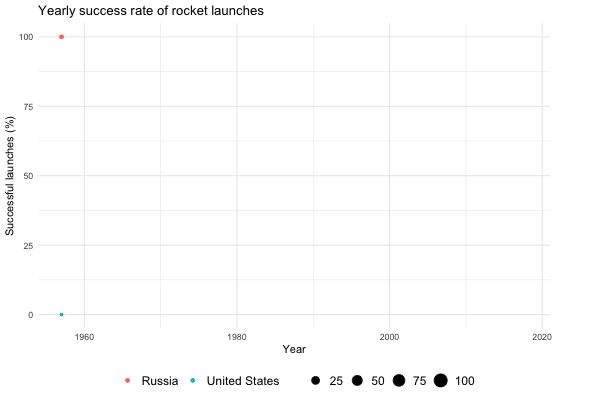Note how the size of the points shrinks and expands. The size is relative to the number of launches for a given year, adding an extra dimension to the visualisation. Also note the abrupt dip in the number of successful American launches in 1986. This is a consequence of the Challenger disaster on January 28, which dealt a significant blow to the US manned space program.

That concludes this week’s Tidy Tuesday. Thanks for reading!